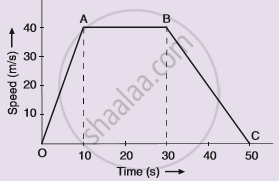# Study the Speed-time Graph of a Car Given Alongside and Answer the Following Questions: - Science

Graph

Study the speed-time graph of a car given alongside and answer the following questions:(i) What type of motion is represented by OA ?
(ii) What type of motion is represented by AB ?
(iii) What type of motion is represented by BC ?
(iv) What is the acceleration of car from O to A ?
(v)What is the acceleration of car from A to B ?
(vi) What is the retardation of car from B to C ?

#### Solution

(i) OA represents uniform acceleration
(ii) AB represents constant speed.
(iii) BC represents uniform retardation.
(iv) Acceleration of car from O to A = slope of line OA

a = ((40-0))/((10-0))
= 4  "m/s"^2
(v) Acceleration of car from A to B is zero as it has uniform speed .
(vi) Retardation of car from B to C = slope of line BC

a = ((40-0))/((50-30)) "m/s"^2
= 2  "m/s"^2

Concept: Velocity - Time Graphs
Is there an error in this question or solution?

#### APPEARS IN

Lakhmir Singh Science Class 9 Physics
Chapter 1 Motion
Hots Questions | Q 62 | Page 44